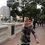# Costa Rica IMO TST 1, Day 2

1. Consider a point $A$ in the interior of a circumference, different from its center. Consider all the chords (except the diameter) that pass through $A$. Find the locus of all the intersection points of the two tangents to the circumference that intersect it on the chords' extremes.

2. Let $s_n$ be a sequence such that $s_1=1$ and $s_{n+1}=3s_n+1$. Note that $s_{18}=193710244$ ends with two identical digits. Prove that all of the sequence's terms that end with two or more identical digits come in groups of three, and that these three terms finish with the same number of identical digits.

3. In a lottery game, a committee picks six different numbers from $1$ to $36$ at random. A ticket consists of six numbers (chosen by you) from $1$ to $36$. A ticket is said to be a "winning ticket" if all of its numbers are different from the numbers picked by the committee. Prove that with 9 tickets you can guarantee having at least one winning ticket, but that with 8 you cannot.Note by José Marín Guzmán
6 years, 4 months ago

This discussion board is a place to discuss our Daily Challenges and the math and science related to those challenges. Explanations are more than just a solution — they should explain the steps and thinking strategies that you used to obtain the solution. Comments should further the discussion of math and science.

When posting on Brilliant:

• Use the emojis to react to an explanation, whether you're congratulating a job well done , or just really confused .
• Ask specific questions about the challenge or the steps in somebody's explanation. Well-posed questions can add a lot to the discussion, but posting "I don't understand!" doesn't help anyone.
• Try to contribute something new to the discussion, whether it is an extension, generalization or other idea related to the challenge.

MarkdownAppears as
*italics* or _italics_ italics
**bold** or __bold__ bold
- bulleted- list
• bulleted
• list
1. numbered2. list
1. numbered
2. list
Note: you must add a full line of space before and after lists for them to show up correctly
paragraph 1paragraph 2

paragraph 1

paragraph 2

[example link](https://brilliant.org)example link
> This is a quote
This is a quote
    # I indented these lines
# 4 spaces, and now they show
# up as a code block.

print "hello world"
# I indented these lines
# 4 spaces, and now they show
# up as a code block.

print "hello world"
MathAppears as
Remember to wrap math in $$ ... $$ or $ ... $ to ensure proper formatting.
2 \times 3 $2 \times 3$
2^{34} $2^{34}$
a_{i-1} $a_{i-1}$
\frac{2}{3} $\frac{2}{3}$
\sqrt{2} $\sqrt{2}$
\sum_{i=1}^3 $\sum_{i=1}^3$
\sin \theta $\sin \theta$
\boxed{123} $\boxed{123}$

Sort by:

Isn't the first one just the polar of A wrt circle. i.e. locus is just a straight line ?

- 6 years, 4 months ago

Yes. I wonder why such a basic fact is presented as a TST problem.

- 6 years, 4 months ago